### 2.3.3.2 Discussion of the Diffusion Approximation

The framework of the diffusion approximation allows to considerably simplify the structure of the transport equations. To point out the implications of the diffusion approximation in this section two important moments are evaluated without this approximation.

Let now be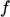a shifted distribution function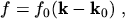(2.127)

where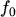is not only symmetric in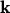but also in every component of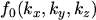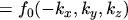(2.128)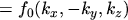(2.129)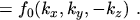(2.130)

This stronger symmetry property ensures that the resulting tensor quantities are of diagonal shape (see eqn. (2.83)). Functions which satisfy this stronger symmetry criterion are for example an isotropic2.7 distribution function whereis only a function of the absolute value of,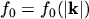, and whose iso-surfaces are spheres. Another example is a distribution with ellipsoidal iso-surfaces, for instance an anisotropic MAXWELL distribution.

As has already been shown in eqns. (2.106) to (2.108), every function can be split into its symmetric and its anti-symmetric part. Since the weight functions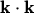and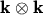are even functions, only the symmetric part of the distribution function has to be taken into account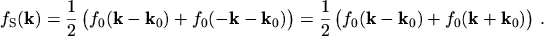(2.131)

Eqn. (2.131) is now used in the evaluation of the statistical average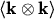: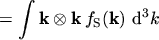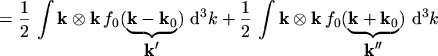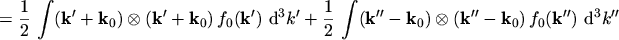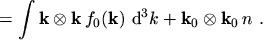(2.132)

Cross terms containing bothand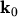vanish because of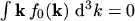.

The statistical average of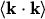can be evaluated in the same way yielding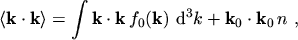(2.133)

For a MAXWELL distribution the first term of the RHS of eqn. (2.133) has already been calculated as eqn. (2.120). Therefore eqn. (2.133) can be written as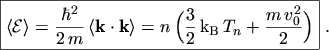(2.134)

As can be seen, without the diffusion approximation the average carrier energy is composed of a thermal component and a kinetic2.8 component. A consequence of the diffusion approximation is that the kinetic term is neglected [24, p.71] [25, p.736]. By assuming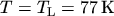,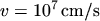, and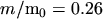for electrons in silicon [9, p.191] the ratio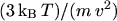yields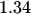. However, in reality this ratio is much bigger because in the regions, where the assumed electron saturation velocity is reached, the electron temperature is much higher than the lattice temperature [26, p.34]. Neglecting the kinetic term appears therefore justified. Note that simulations at very low temperatures would have to include this term. Under dynamic conditions this term can also be significant [27, p.413].

The first term of the RHS of eqn. (2.132) has also already been calculated as eqn. (2.88). Eqn. (2.132) can therefore be written as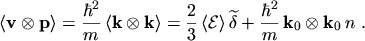(2.135)

Inserting eqn. (2.135) into eqn. (2.76) yields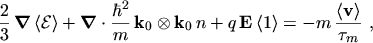(2.136)

By calculating the statistical average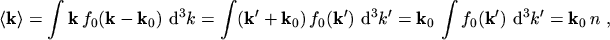(2.137)

the current density can be expressed as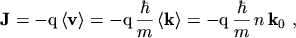(2.138)

and the second term of the LHS of eqn. (2.136) can be written as(2.139)

where in the last identity the term containing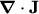has been neglected.

Inserting eqn. (2.139) into eqn. (2.136) yields the final form of the current relation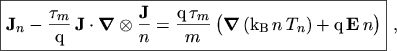(2.140)

which has compared to eqn. (2.102) an additional second term  on the LHS, which is nonlinear in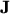. This example demonstrates that neglection of the term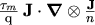is another consequence of the diffusion approximation. Therefore, if it is justified to neglect the kinetic term in eqn. (2.134) it is equally valid to neglect the additional term in the current equation (2.140).

The importance of this additional term within semiconductor equations is controversial. Phenomena known from fluid dynamics like super-sonic transport and propagation of electron shock-waves arise  . The resulting transport model is referred to as (full) hydrodynamic transport model2.9.

M. Gritsch: Numerical Modeling of Silicon-on-Insulator MOSFETs PDF